Zero Exponents

Look at the Quotient of a Power Rule,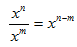, and consider what happens when n = m. When n = m, n - m = 0 and the expression would be x0. What does the exponent of zero mean? The following example will explain.

Example Simplify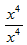Using expanded notation: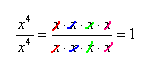Using the Quotient of a Power Rule: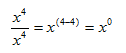Therefore, x0 must equal 1.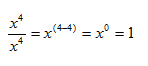This works for any value of the base except when it is 0, because an exponent on zero would mean that zero would multiply itself a certain number of times. A product with zero is always zero. This leads to the next power rule.

Zero Exponent Rule :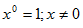.